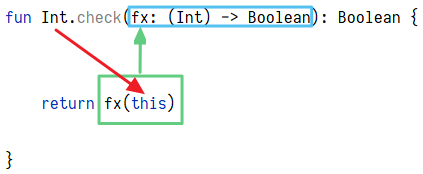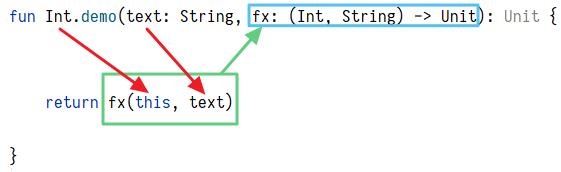### [Kotlin]研究 Kotlin 的函式宣告、函式型別、匿名函式、Lambda 與高階函式

Kotlin 的「函式」是所謂的「一級函式」，支援「高階函式」的用法，也可宣告「匿名函式」及「巢狀函式」，這些都是近幾年所興起的程式語言特色。

(原本這篇文章只是要整理一下 Kotlin 的函式用法而已，沒想到愈寫愈多!!)

## 名詞定義

### Expression (表達式、表示式、運算式)

• 它是一種「值」
• 單獨存在沒有意義
• 可放在「等號」的右邊
• 可做為函式的引數 (Argument)
• 可做為函式的傳回值
• 例如: 數值、字串、布林值、null、運算後的結果、比較後的結果、匿名函式...

### 其它名詞定義

• Literal: 字面值，例如: 103.14truenull'A'"This is a book"...
• Parameter: 參數，函式「宣告」時所輸入的值，例如: fun example(參數) { }
• Argument: 引數，函式「執行」時所引用的值，例如: example(引數)
• Identifier: 識別字，命名變數、函式、類別...時所使用的文字
• Lambda: 一種匿名函式的寫法或概念

## Kotlin 函式種類

B.單一表達式 fun f()= 一行 函式 隱性傳回

B.單一表達式 fun()= 一行 物件 隱性傳回
C.Lambda {->} 可多行 物件 隱性傳回

~ 物件 ~

• 標記紅色字為函式原本就會使用到的「參數及型別」、藍色字為「函式主體」。

### 具名函式

#### A. 敘述式:

• 重點: 以 fun 開頭、函式主體有多行、寫 return 傳回
```fun sum (m:Int, n:Int): String {
return "Result: \${m + n}"
}```

#### B. 單一表達式:

• 重點: 以 fun 開頭、函式主體只能有一行、隱性傳回最後的值
`fun sum (m:Int, n:Int): String = "Result: \${m + n}"`

#### 呼叫函式與傳遞引數:

`println(sum(1, 2)) // Result: 3`

### 匿名函式 (物件)

• 把具名函式裡的函式名稱拿掉
• 好比是「工具人」，把事情做完就可以走了
• 所有的匿名函式都是「表達式」
• 匿名函式的本質是「物件」

#### A. 仿敘述式:

• 重點: 函式主體有多行、寫 return 傳回
```fun(m:Int, n:Int): String {
return "Result: \${m + n}"
}```

#### B. 單一表達式:

• 重點: 函式主體只能有一行、隱性傳回最後的值
`fun(m:Int, n:Int): String = "Result: \${m + n}"`

#### C. Lambda:

• 重點: 僅用大括號來表現函式、函式主體有多行、隱性傳回最後的值、可用 it 取代唯一參數
```{ m:Int, n:Int ->
"Result: \${m + n}"
}```

### 函式物件

#### 函式型別:

Kotlin 是一種「強型別」語言，每一種值都有它的「資料型別」，因此函式物件也有屬於自己的型別，稱之為「函式型別」。

```var 變數名稱: 資料型別
var 變數名稱: 資料型別 = 值```

```var 函式名稱: 函式型別
var 函式名稱: 函式型別 = 匿名函式```

「函式型別」的宣告比較複雜! 因為要識別整個匿名函式的「輸入型別」及「輸出型別」，所以寫做 (輸入型別)->輸出型別。若該匿名函式沒有輸入也沒有輸出則寫做 ()->Unit

• 標記紫色字為「函式型別」

#### A. 仿敘述式:

```var sum: (Int, Int) -> String = fun(m:Int, n:Int): String {
return "Result: \${m + n}"
}
// 或是省略函式型別:
var sum = fun(m:Int, n:Int): String {
return "Result: \${m + n}"
}```

#### B. 單一表達式:

```var sum: (Int, Int) -> String =
fun(m:Int, n:Int): String = "Result: \${m + n}"
// 或是省略函式型別:
var sum =
fun(m:Int, n:Int): String = "Result: \${m + n}"```

#### C. Lambda:

```var sum: (Int, Int) -> String = { m: Int, n: Int ->
"Result: \${m + n}"
}
// 或是省略函式型別:
var sum = { m: Int, n: Int ->
"Result: \${m + n}"
}```

#### 呼叫函式與傳遞引數:

`println(sum.invoke(1, 2)) // Result: 3`

`println(sum(1, 2)) // Result: 3`

#### 函式名稱衝突:

Kotlin 允許相同名稱的「具名函式」與「函式物件」同時存在，但具名函式的優先權高於函式物件。執行 functionName() 會呼叫「具名函式」，此時若要呼叫「函式物件」，必須用函式物件原本的 invoke() 方法:

`functionName.invoke()`

#### 其它用法:

1. 若 Lambda 函式只有一個輸入參數，可用 it 取代:

```var inc = { n: Int ->
"Result: \${n + 1}"
}
// 簡化成:
var inc = {
"Result: \${it + 1}"
}
println(inc(2)) // Result: 3```

2. 巢狀 Lambda:

```var sum: (Int, Int) -> (Int, Int) -> String = { m: Int, n: Int ->
{ x: Int, y: Int ->
"Result: \${m + n + x + y}"
}
}
// 或是省略函式型別:
var sum = { m: Int, n: Int ->
{ x: Int, y: Int ->
"Result: \${m + n + x + y}"
}
}
println(sum(1, 2)(3, 4)) // Result: 10```

3. 可用 :: 將「具名函式」轉成「函式物件」:

```// 宣告具名函式 oldSum():
fun oldSum(m:Int, n:Int): String {
return "Result: \${m + n}"
}
println((::oldSum)(1, 2)) // Result: 3
// 將 oldSum() 轉成函式物件:
var sum = ::oldSum
println(sum(1, 2)) // Result: 3
```

## 高階函式

Kotlin 支援所謂的「高階函式」，意思是函式可以作為「參數」傳入另一個函式，也可以被函式作為「傳回值」，而這些特性通常與「匿名函式」與「函式物件」相關。

### 範例 1

• 說明: 因為以下的範例是為了「擴充」既有的型別，原本的「整數值」即為函式的第一個參數，在函式中是用 this 取代。而後面傳入的匿名函式其實是第二個參數。
• 標記紅字為「匿名函式」。
```// 函式宣告
fun Int.check(fx: (Int) -> Boolean): Boolean {
return fx(this)
}```

#### 不專業的圖解:```// 使用匿名函式，判斷整數 7 是否大於 9
// 1. 仿陳述式
println(7.check(fun(n: Int): Boolean { return n > 9 })) // false
// 2. 使用表達式
println(7.check(fun(n: Int) = n > 9)) // false
// 3. 使用 Lambda
println(7.check({ n: Int -> n > 9 })) // false
```

#### Lambda 簡化:

1. 可用 it 取代唯一參數
2. 若 Lambda 為最後一個引數，可提取至小括號的後面
3. 若 Lamba 為唯一的引數，可省略小括號

```println(7.check({ it > 9 })) // false
println(7.check() { it > 9 }) // false
println(7.check { it > 9 }) // false```

#### 高階函式的好處:

```println(7.check { it % 2 == 0 }) // 判斷整數 7 是否為偶數
println(12.check { it in 10..100 }) // 判斷整數 12 是否介於 10 ~ 100```

### 範例 2

```// 函式宣告
fun Int.demo(text: String, fx: (Int, String) -> Unit): Unit {
return fx(this, text)
}```

#### 不專業的圖解:```// 用 Lambda 傳入的執行結果
5.demo("cars", { n, text -> println("The number of \$text is \$n") }) // The number of cars is 5
9.demo("cars") { n, text -> println("The number of \$text is \$n") } // The number of cars is 9```

## 不專業的結論

• 在寫程式時，我們通常會將「具名函式」宣告在程式的獨立區塊，即 main() 的外面，會優先被載入記憶體。
• 「匿名函式」與「函式物件」是宣告在程式要用到的地方，用到時才會建立物件、載入記憶體。
• 多用 Lambda 來寫函式，因為 Lambda 具有多行、簡潔、參數明確的優點。
• 看到「冒號」就會聯想到型別，只要「等號」右邊的型別有宣告清楚，那「等號」左邊的型別可以省略。